# Putting Things in Order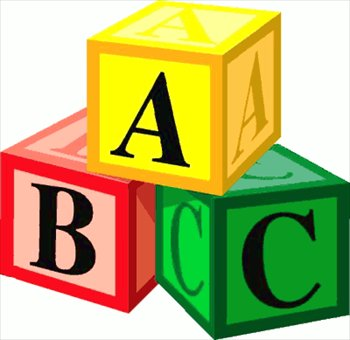Alice the cow loves putting things in order. She plays with her alphabet blocks and she plays with numbers, sorting them into different orders. She even sorts the other cows at the farm into different orders.

There are a few different orders that Alice likes the best. They are increasing and decreasing orders (for numbers), and alphabetical and reverse alphabetical orders for letters. She sorts the cows into different orders, depending on things like their size, the amount of milk they produce, how old they are or how much grass they have eaten. There are many different ways to place objects in order. In this article, we shall explore some of Alice's favourite ordering techniques.

## Ordering Numbers

There are two main ways we can order numbers. These are increasing and decreasing orders.

### Increasing Order

In increasing order, the smallest number comes first, followed by the next smallest number, followed by the next smallest number, and so on. The largest number comes last.

For example, if we were asked to sort the numbers $19,7,2,6,9$ and $3$ into increasing order, we would come up with the list:

$2,3,6,7,9,19$.
Increasing order is the order that we use when we are counting. Each number we say gets larger as we count on.

Let's try one more example. Suppose someone asks you to sort the numbers $23,7,53,28,19$ and $124$ into increasing order. Well, when we count, the first of these numbers that we say is $7$, so it comes first. The next is $19$, so it comes next in our list. The next number we say is $23$, then comes $28$, next comes $53$, and last number we say is $124$. So, the sorted list is

$7,19,23,28,53,124$.

### Decreasing Order

Decreasing order is the opposite of increasing order. If a list of numbers is in decreasing order, they get smaller as you move along the list. For example, the list

$19, 9,7,6,3,2$
is in decreasing order. The largest number comes first, followed by the second largest number, all the way down to the smallest number.

Decreasing order is the opposite of the order you count in. When rockets blast off, the numbers in the count down are said in decreasing order:

$10 \dots 9 \dots 8 \dots 7 \dots 6 \dots 5 \dots 4 \dots 3 \dots 2 \dots 1 \dots 0 \dots \text{ Blast Off!}$For example, if someone asked you to put the numbers $15,3,18,7,2$ and $34$ in decreasing order, you'd start with the biggest, which is $34$, then write down the next biggest, which is $18$, then the next biggest, $7$, followed by the second smallest, which is $3$, and the smallest, which is $2$. So, in decreasing order, the numbers are:

$34,18,15,7,3,2$.

### Ordering Objects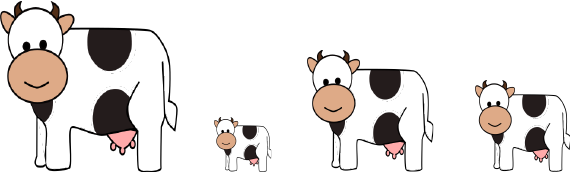Let's see how Alice puts the other cows on the farm into order. For example, she might give each cow a number, based on their weight, height, birthday or the amount of grass they have eaten.

She then sorts the numbers into increasing or decreasing order, and puts the cows in the positions corresponding to their numbers. For example, the picture shows the other cows sorted into decreasing order by their height.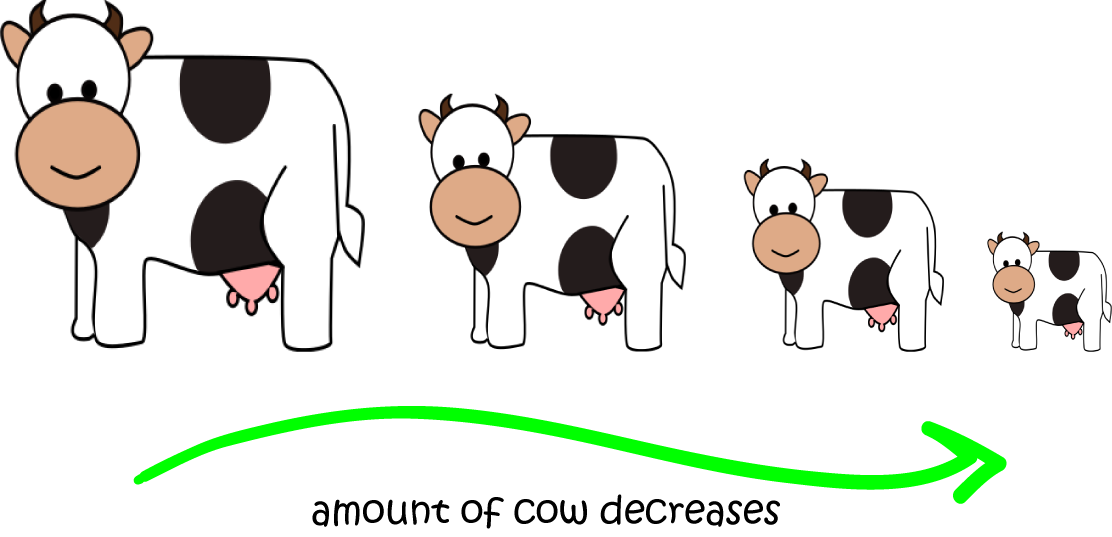You can do the same thing with other objects. For example, the pictures below have been sorted into increasing order by the amount the balloon has been blown up.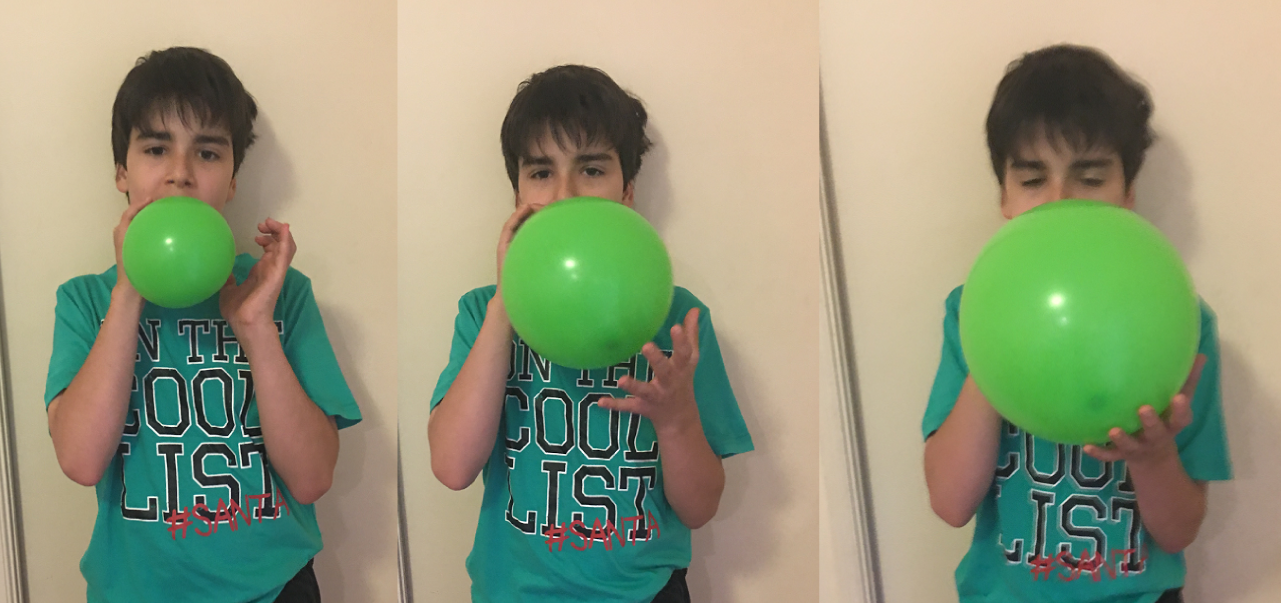You might also sort events into increasing or decreasing order by the time when they happened.

## Ordering Letters and Words

The letters of the alphabet have a special order that we use when we recite the alphabet or sing the alphabet song.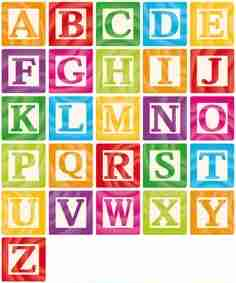We call this alphabetical order or dictionary order.

We can use alphabetical order to put letters, or even words, into order. We put the letters into they order in which they would appear in the alphabet. Putting words into alphabetical order is slightly more complicated, but for now, we'll just look at examples in which we put them in alphabetical order according to their first letters. If two words start with the same letter, the word whose second letter comes first in the alphabet is the one you put first.

For example, the letters G, A, B, F and N can be put into alphabetical order as follows:

• A appears first in the alphabet, so we put it first.
• B is next in the alphabet, so we put it second.
• F comes next in the alphabet, so we put it third.
• G comes next in the alphabet, so we put it fourth.
• N is the last of these letters to appear in the alphabet, so we put it fifth.
So, in alphabetical order, the letters are:
A, B, F, G, N

For example, we can put the words "cow", "frog", "bat", "snail" and "anteater" into alphabetical order as follows:

• These words all start with different letters, so put their first letters into alphabetical order. Their first letters are C, F, B, S and A.
• A appears first in the alphabet, so we put "anteater" first.
• B is next in the alphabet, so we put "bat" second.
• C comes next in the alphabet, so we put "cow" third.
• F comes next in the alphabet, so we put "frog" fourth.
• S is the last of these letters to appear in the alphabet, so we put "snail" fifth.
So, in alphabetical order, the words are:
anteater, bat, cow, frog, snail

You can also put words and letters into reverse alphabetical order. This is just the opposite order to alphabetical order: the letter that appears last in the alphabet goes first and the letter that appears first in the alphabet goes last.

For example, the letters G, A, B, F, and N can be put into reverse alphabetical order as follows:

• A appears first in the alphabet, so we put it last.
• B is next in the alphabet, so we put it second last.
• F comes next in the alphabet, so we put it third last.
• G comes next in the alphabet, so we put it second.
• N is the last of these letters to appear in the alphabet, so we put it first.
So, the letters can be listed in reverse alphabetical order as follows:
N, G, F, B, A

## Conclusion

Being able to put numbers, letters, words and objects into order is a very useful skill, and one that you should practise.

Why not try putting the first letters of your friends' names into alphabetical order and reverse alphabetical order? You could also try ordering your friends by their heights, or their birthdays.

Something else to try is putting bus numbers into increasing or decreasing order.

Why not try to come up with some strange objects that you can put in order, and some different ways of ordering them? As Alice the cow will tell you, putting things in order is lots of fun!

### Description

This mini book covers the core of Math for Foundation, Grade 1 and Grade 2 mathematics including

1. Numbers
3. Subtraction
4. Division
5. Algebra
6. Geometry
7. Data
8. Estimation
9. Probability/Chance
10. Measurement
11. Time
12. Money
13. and much more

This material is provided free of cost for Parent looking for some tricks for their Prekinder, Kinder, Prep, Year 1 and Year 2 children

### Learning Objectives

These lessons are for kids aged 4-8 with the core objective to expose their brains to concepts of addition, subtraction, division, algebra and much more.

Author: Subject Coach
You must be logged in as Student to ask a Question.